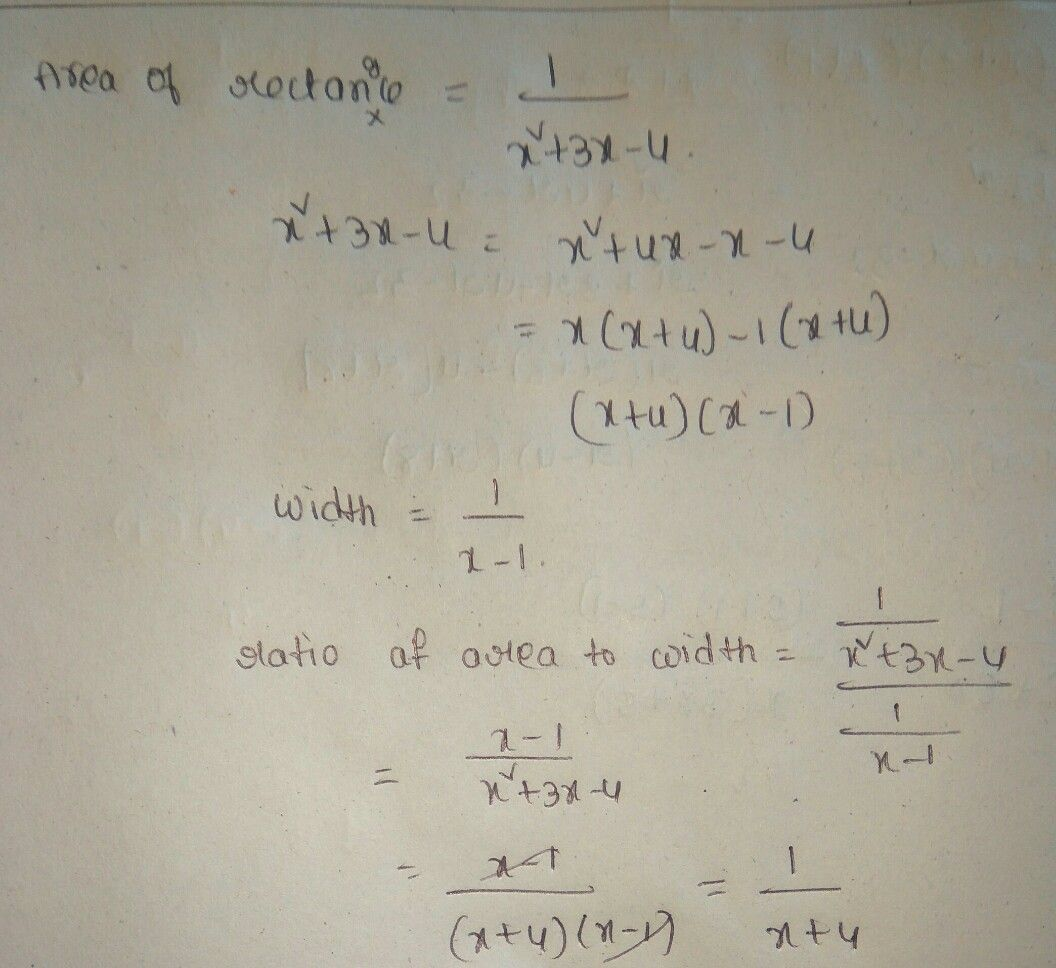Symbol
Problem$1$ $3$ The area of a rectangle is $x^{2+3x-4}$ . The width of the rectangle is $\dfrac {1} {x-1}$ Determine the ratio of the area to the width.
7th-9th grade
Algebra
Search count: 106
SolutionQanda teacher - saikumarpls provide extra gift coins